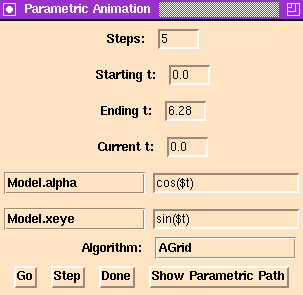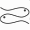# The Parametric Animation Panel### The Pisces Parametric Animation Panel.

The parametric animation panel can animate a two-parameter family of model along a one-parameter path in parameter space. In other words, if a model depends on the parameters (a,b), then the parametric animation panel allows the user to animate level sets of the model along any path (a(t), b(t)).

## How it works

The animation is created by the following algorithm:
• The step size is computed by taking the difference between Ending t and Starting t and dividing the difference by the Steps.
• The current values values of the parameters to animate are saved. We will denote these parameters as (a,b).
• The value of Current t is set to the value of Starting t. This value and the Tcl expressions next to the parameters to animate are used to determine the point (a(t0), b(t0)) which will be used as the parameter values for the initial level set.
• The Algorithm is called to compute the level set.
• The value of Current t is incremented by step size.
• If the value of Current t is less then or equal to the Ending t then go to the previous step.
• The value of the animation parameters are restored.

## Controlling the Algorithm from Pisces

The parametric animation panel has the following fields:
Steps
The number of frames of animation that will be produced. This must be a positive integer.
Starting t
The value that the independent variable takes for the first frame of the animation.
Ending t
The value the independent variable takes for the last frame of the animation.
Current t
The current value of the independent variable.
Variable/Expression
The menus and Tcl expression in these fields determine the parameters to animate and determine the parametric paths that the parameters will follow. The main point to remember about Tcl expressions is that you must place a \$ (dollar sign) in front of the name of the variable (which is always t).
Algorithm
This menu selection determines method of computing the level set. The parameters for each algorithm may be set using the control panel specific to each algorithm.
To see the animation press the Go button. To step through the animation one frame at a time, you may press the Step button.

The animation path you have specified may be viewed by pressing the Show Parametric Path button.

### Known Bugs

Same as for the animate panel. Also, the name of the independent variable must be t.

### Bug Reports

software@geom.umn.edu

### Implemented By

John Golden and Erik Streed.
Next: The Parametric Animation Panel
Previous: User InterfaceThe Pisces Home Page